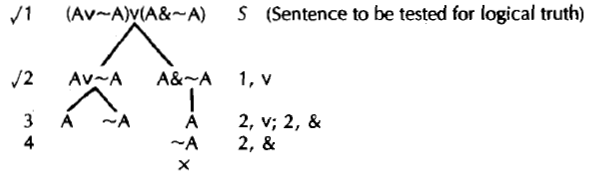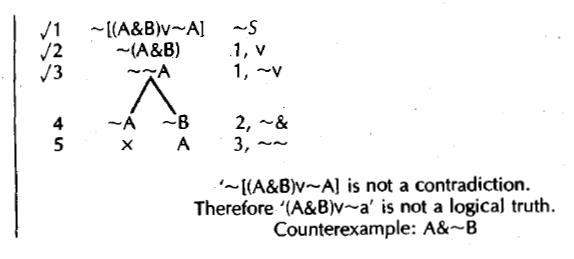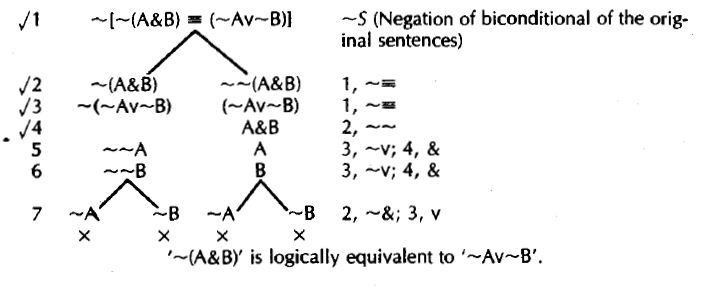# 9.2: Other Uses for Truth Trees

$$\newcommand{\vecs}{\overset { \rightharpoonup} {\mathbf{#1}} }$$ $$\newcommand{\vecd}{\overset{-\!-\!\rightharpoonup}{\vphantom{a}\smash {#1}}}$$$$\newcommand{\id}{\mathrm{id}}$$ $$\newcommand{\Span}{\mathrm{span}}$$ $$\newcommand{\kernel}{\mathrm{null}\,}$$ $$\newcommand{\range}{\mathrm{range}\,}$$ $$\newcommand{\RealPart}{\mathrm{Re}}$$ $$\newcommand{\ImaginaryPart}{\mathrm{Im}}$$ $$\newcommand{\Argument}{\mathrm{Arg}}$$ $$\newcommand{\norm}{\| #1 \|}$$ $$\newcommand{\inner}{\langle #1, #2 \rangle}$$ $$\newcommand{\Span}{\mathrm{span}}$$ $$\newcommand{\id}{\mathrm{id}}$$ $$\newcommand{\Span}{\mathrm{span}}$$ $$\newcommand{\kernel}{\mathrm{null}\,}$$ $$\newcommand{\range}{\mathrm{range}\,}$$ $$\newcommand{\RealPart}{\mathrm{Re}}$$ $$\newcommand{\ImaginaryPart}{\mathrm{Im}}$$ $$\newcommand{\Argument}{\mathrm{Arg}}$$ $$\newcommand{\norm}{\| #1 \|}$$ $$\newcommand{\inner}{\langle #1, #2 \rangle}$$ $$\newcommand{\Span}{\mathrm{span}}$$

So far we have used truth trees exclusively to test the validity of arguments. But now that we understand how truth trees work we can easily apply them to do all sorts of other jobs. For example, suppose I hand you the sentence '~(AvB)&(~A⊃B)' and ask you whether it is a contradiction. You could always work this problem with truth tables. If in all lines of the truth table the sentence is false, it is, by definition, a contradiction. If the sentence is true in one or more cases it is not a contradiction. But a truth tree will get the job done faster. If we make a sentence the initial line on a truth tree, we know the truth tree will find us a case in which the sentence is true, if there is such a case. If there is no such case, all paths on the tree will close. Can you see how the truth tree will tell us whether the sentence is a contradiction?

To explain this more thoroughly, we can again use the idea of a counterexample. A contradiction is false in all cases. So a case in which a sentence is true constitutes a Counterexample to its being a contradiction. The truth tree method applies immediately to look for counterexamples to a sentence being a contradiction. We make the sentence to be tested the first line of a tree. If there are one or more counterexamples, that is, cases in which the sentence is true, the tree method is guaranteed to find them. If the tree method does not turn up a counterexample, that is, if all paths close, we know there are no cases in which the sentence is true. But if there are no cases in which it is true, the sentence is false in all cases; in other words, it is a contradiction. We can summarize this test by saying

To test a sentence for being a contradiction, make the sentence the first line of a truth tree. If there is an open path in the tree, this path provides a counterexample to the sentence being a contradiction. If all paths close, the sentence is a contradiction.

Applying this test to our example we getIs the sentence 'A≡(~AvC)' a contradiction?Note that I have written down the result of my test, that the sentence to be tested is not a contradiction. And note how I also put down the counterexample which shows this.

A final small point about this example. In the annotation for line 4, 1 have listed two rules. This is because I applied the rule v to the disjunction on the left branch of line 3, and I separately applied the rule ~V to the separate sentence of line 3 on the right branch.

Can we use truth trees to determine whether a given sentence is a logical truth? Sometimes students propose the following test for a sentence being a logical truth: List the sentence and see if all branches remain open. Close, but no cigari The proposed test mistakenly tells us that 'A' is a logical truth. If we make 'A' the initial line of a tree, there is nothing to do, and all branches are open. But 'A' is not a logical truth. We also get the wrong answer if we apply the proposed test to (Av~A)v(A&~A):One branch of this tree does close. But the initial sentence is a logical truth, as you can tell from the fact that one of its disjuncts is a logical truth.

However, there is a very simple way to use the tree method to test for a logical truth. Just use the test for contradictions? How? A sentence is a logical truth just in case it is true in all cases. But a sentence is true in all cases just in case its negation is false in all cases. So a sentence is a logical truth if and only if its negation is a contradiction. Suppose, now, that I ask you whether a sentence is a logical truth, for example, the sentence of the very last example. Take the negation of the sentence. Determine whether this negation is a contradiction. If the negation is a contradiction, then the original sentence was a logical truth. If the negation is not a contradiction, then the original sentence was not a logical truth:Notice that this last tree is an example of a completed tree in which not all compound sentences have been checked. I never worked on line 3 because all branches closed before I got to line 3. Once all the branches close, the tree is finished. There is no way to make all the initial sentences true. If any sentences have not been worked when all branches close, continuing and working them would give us no new information.

Let us similarly test '(A&B)v~A' to see whether it is a logical truth:How should we understand the counterexample here? The case A&~B ('A' true and 'B' false) is a case in which '~[(A&B)v~A]', the sentence tested for being a contradiction, is true. But '~[(A&B)v~A]' is true in a case if and only if '(A&B)v~A', the sentence tested for being a logical truth, is false in the case. A case in which a sentence is f&e proves that the sentence is not a logical truth. Such a case constitutes a counterexample to the sentence being a logical truth. So the case A&~B is a counterexample to '(A&B)v~A' being a logical truth. Clearly, this will hold generally:

For any sentence X, any case which is a counterexample to ~X being a contradiction will also be a counterexample to X being a logical truth.

To summarize the test for logical truth:

To test a sentence for being a logical truth, make the negation of the sentence the first line of a truth tree. If d the paths close, the sentence is a logical truth. An open path gives a counterexample to the original sentence being a logical truth.

This summary makes no mention of the intermediate role of a test for contradiction. If you do not understand the test as just summarized, go back over the last two examples and make sure you can see how they fit in with the summary I have just given.

We can do yet more with the truth tree method. Recall that two sentences are logically equivalent just in case their biconditional is a logical truth. Thus we can use the test we have just devised for logical truth to determine whether two sentences are logically equivalent:

To test whether X and Y are logically equivalent, test X≡Y for being a logical truth. If X≡Y is a logical truth, X and Y are logically equivalent. If X≡Y is not a logical truth, X and Y are not logically equivalent. A counterexample to X≡Y being a logical truth is also a counterexample to the logic equivalence of X and Y. That is, it is a case in which one of the two sentences, X and Y, is true and the other is false.

Can you see why a counterexample to the logical truth of X≡Y is also a case in which one of the two sentences, X and Y, is true and the other is false? A counterexample to X≡Y being a logical truth is a case in which X≡Y is false. But a biconditional is false if and only if one of its components is true and the other is false, that is, if X is true and Y is false or the other way around. Finally, you can see why we would call such a case a counterexample to the logical equivalence of X and Y. X and Y are logically equivalent if and only if in all cases they have the same truth value. So if we have come up with a case in which one sentence is true and the other is false, we have a case which proves by counterexample that they are not logically equivalent.

To illustrate this method of determining logical equivalence, I will use it to verify one of De Morgan's rules. To show: '~(A&B)' is logically equivalent to '~Av~B'.This section will introduce you to one more notion:

A set of one or more sentence logic sentences is consistent if and only if there is at least one assignment of truth values to sentence letters which makes all of the sentences true.

The truth tree method applies immediately to test a set of sentences for consistency. Before reading on, see if you can figure out this test for yourself.

The tree method works by finding a case in which all initial sentences on a tree are true, if there is such a case. So, to determine whether the sentences in a given set are consistent, list the sentences as the initial part of a tree. If there is a case in which all of these sentences are true together, the tree method will find it. Such a case constitutes what logicians call a Model. which shows the initial sentences to constitute a consistent set. If the tree closes, the set of sentences has no model and is Inconsistent:

A Model of a set of sentence logic sentences is an assignment of truth values to sentence letters which makes all of the sentences in the set true.

To test a finite set of sentences for consistency, make the sentence or sentences in the set the initial sentences of a tree. If the tree closes, there is no assignment of truth values to sentence letters which makes all the sentences true (there is no model), and the set is inconsistent. An open branch gives a model and shows the set to be consistent.

To make the statement of the test correct, I have had to use the notion of a Finite Set of sentences, that is, a set or collection of a limited, as opposed to an unlimited, number of sentences. You do not need to understand the distinction between a finite as opposed to an infinite set to understand anything in Volume I and Volume 11, Part I of this text. But you may explore the idea and its implications for consistency by working through exercise 9-7.
It's time to practice these new applications of truth trees.

Exercise

9-3. Use the truth tree method to determine which of the following sentences are logical truths. Show your completed trees. If a sentence is not a logical truth, give the counterexample or counterexamples which show this.

a) ~(~F&G)v~(F&G)

b) [H⊃(O⊃N)]⊃[(H&O)⊃N]

c) [~S&(GvK)]v[~G⊃(SvK)]

d) [(FvG)⊃(P&Q)]⊃(~Q⊃~F)

e) [Lv(M&N)]⊃(LvM)&N]

f) [(K&M)&P]⊃[(~K&P)v(Kv~M)]

g) [L⊃(M⊃N)]⊃[(L⊃M)⊃(L⊃N)]

h) [(SvT)⊃F]⊃{[(FvG)⊃H]⊃(S⊃H)}

i) (I&~J)v[(J&K)v~(K&I)]

j) {[C&(AvD)]v~(C&F)}v~(A&~G)

9-4. Use the truth tree method to determine which of the following sentences are contradictions. Show your completed trees, and for each sentence you determine not to be a contradiction, give the counterexample or counterexamples which show this.

a) (A&B)&(~Av~B)

b) (FvG)&(~Fv~G)

c) (FvG)≡(~F&~G)

d) [Iv(J&K)]⊃[(IvJ)&K]

e) [(H&F)⊃C]&~[H⊃(F⊃C)]

f) ~{[B&(Mv~P)]⊃[P⊃(~Mv~B)]}

g) (A≡B)≡(~A≡B)

h) [(~FvQ)v(P&Q)&~(~Q⊃~F)

i) [K⊃(G⊃P)&[(~KvD)&~(K⊃P)]

j) [(~GvQ)&(K⊃G)]&~(Pv~K)

9-5. Use the information suggested in exercise 4-3 to state a new truth tree test for logical equivalence. Comment on the relation between this test and the test given in the text.

9-6. Use the truth tree method to determine which of the following pairs of sentences are logically equivalent. You may use either the test given in the text or the closely related test you discovered in exercise 9-4. Show your completed trees, and when you find a pair which is not logically equivalent, give the counterexample or counterexamples which show this.

a) A⊃~A and ~A

b) ~(lvJ) and ~I&~J

c) Mv~H and ~M⊃H

d) ~(F&P) and ~F&~P

e) (D&N)⊃J and D⊃(N⊃J)

f) (I⊃Q)⊃D and l⊃(Q⊃D)

g) L&(SvT) and (L&S)v(L&T)

h) Hv~(~Pv~Q) and (HvP)&(HvQ)

9-7. Consider the following definition:

(C1) A sentence of sentence logic is consistent if and only if it is not a contradiction.

a) Are all logical truths consistent according to definition (C1)? Explain why or why not.

b) Show that a sentence logic sentence is consistent according to definition (C1) if and only if there is at least one assignment of truth values to sentence letters which makes the sentence true.

If you go on in your study of logic, the more general notion of consistency already given in the text will turn out to be very important:

(C2) A set of one or more sentence logic sentences is consistent if and only if there is at least one assignment of truth values to sentence letters which makes all of the sentences true.

c) Show that a set of sentences is consistent according to definition (C2) if and only if the conjunction of all the sentences in the set is consistent according to definition (C1).

This last problem seems to show that the two definitions of consistency come to the same thing. Why, then, did I say that the second definition is more general? Actually, there is something not quite right about exercise (c). To see what this is, you need to understand the difference between a finite and an infinite set of sentences. A finite set of sentences has some definite number of sentences in it, such as 2, or 47, or 1,007,859. In an infinite set of sentences the list of sentences goes on without end. There is no integer which gives you the number of sentences in an infinite set.

d) Here is an example of an infinite set of sentences:

~A, ~~A, ~~~A, ~~~~A, ~~~~~A, . . .

The first sentence is '~A'. The second sentence is '~~A'. The third, fourth, fifth, and further sentences are 'A' preceded by 3, 4, 5, and further negation signs, so that the list goes on forever. Question: Is this set consistent?

The difficulty with exercise (c) is that it makes sense only if you assume that the set of sentences is finite. For if the set is infinite, there is no sentence which is the conjunction of all its members. This is because in sentence logic, all sentences are finite in length.

Now you can see why definition (C2) is more general than (C1). (C2) gives sense to the consistency of an infinite set of sentences. The two definitions really come to the same thing, but only for finite sets of sentences.

e) Describe a consistent infinite set of sentences.

f) Use the truth tree method to test the following sets of sentences for consistency. In each case, show your tree. Write next to your tree whether the set is consistent or inconsistent, and when consistent, give all the models for the set which the truth tree provides.

f1) PvS, P⊃S

f2) (~F⊃S)>F, ~F, S

f3) K&[(~K&H)vH]

f4) NvB, Nv~B, -NvB

From a logical point of view, you should really think of the truth tree method as a method for testing a set of sentences for consistency. This general method then has more specific applications, such as testing an argument for validity. This is because

An argument is valid if and only if the set comprised by the argument's premises and the negation of its conclusion is inconsistent.

We say the same thing in other words by saying that an argument is valid if and only if the negation of the conclusion is inconsistent with (the set of) the argument's premises.

g) Explain why the last offset statement is correct.

Some textbooks first present the truth tree method as a test of consistency and then apply it to argument validity. I introduced trees as a test for argument validity because I wanted to motivate the introduction of trees with something you already know about, namely, arguments. It is initially hard for many students to understand the interest in consistency and inconsistency, but these notions will become very important in Volume 11, Part I1 of the text.

chapter summary Exercise

Here are the important terms and ideas from this chapter. Write your explanations for them as usual. This list repeats some terms from previous chapters.

a) Main Connective

b) Logical Truth

c) Truth Tree Test for Logical Truths

e) Truth Tree Test for Contradictions

f) Logical Equivalence

g) Truth Tree Test for Logical Equivalence

h) Consistency

i) Model

j) Infinite Set of Sentences

k) Truth Tree Test for Consistency of a Finite Set of Sentences

9.2: Other Uses for Truth Trees is shared under a not declared license and was authored, remixed, and/or curated by LibreTexts.